# Air core inductor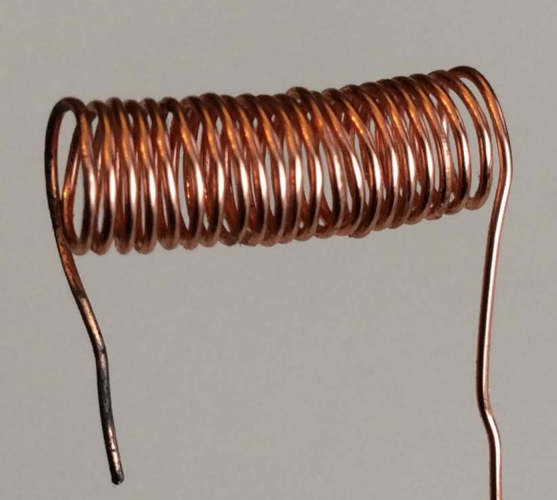Air core inductor

Air core inductors that consist of a coil of conducting wire with no core. They are used in all sorts of electronic devices like radios and computers.

### Formulas

The following formulas can be used to calculate the inductance, length, diameter and number of turns of an air core inductor and the total length of wire used.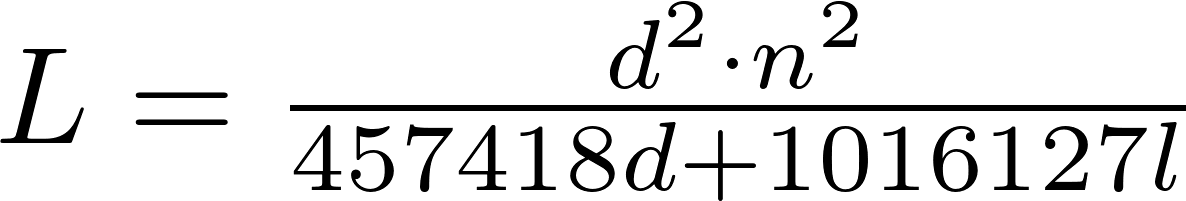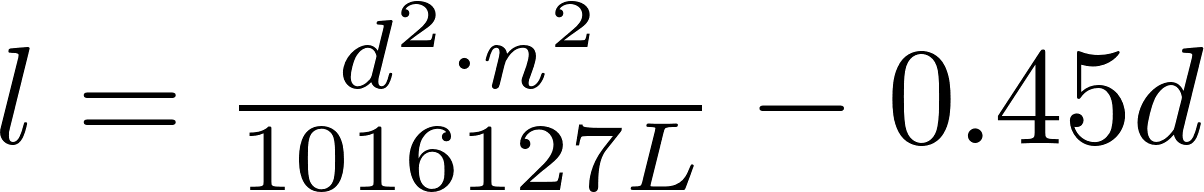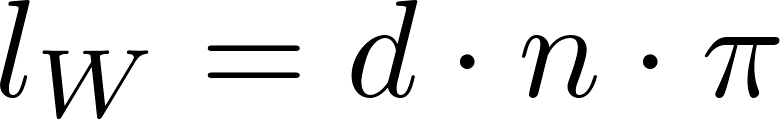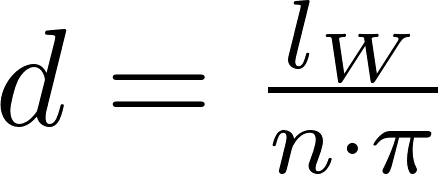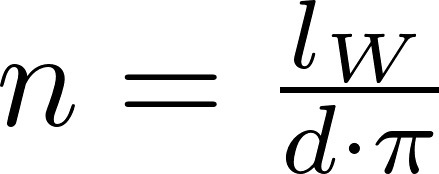L is the symbol for inductance and is measured in henry (H).
l is the symbol for length and is measured in meter (m).
d is the symbol for diameter and is measured in meter (m).
n is the number of turns
l W is the symbol for wire length and is measured in meter (m).